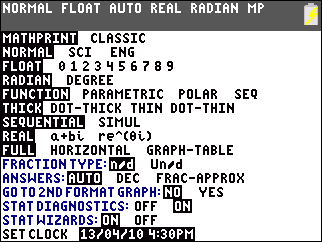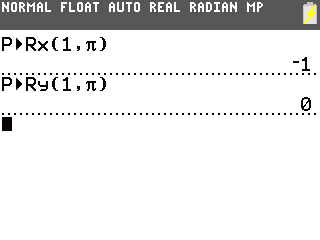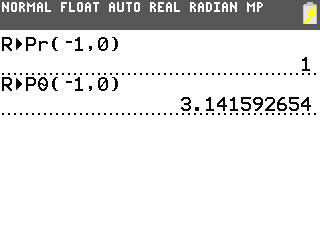# Knowledge Base

## Solution 34507: Performing Polar and Rectangular Conversions on the TI-83 Plus and TI-84 Plus Family of Graphing Calculators.

### How do I perform polar and rectangular conversions on the TI-83 Plus and TI-84 Plus family of graphing calculators?

The examples below demonstrate how to perform polar to rectangular and rectangular to polar coordinate conversions. Converting coordinates requires two separate operations, one for each point in an ordered pair.

For Example: Convert polar coordinates (1, p) to rectangular coordinates using P►Rx( and P►Ry(

1) Press [MODE].
2) Press [Down Arrow] multiple times until "RADIAN" is selected.
3) Press [ENTER] [2ND] [QUIT].4) Press [2ND] [ANGLE] .
5) Press  [ , ] [2ND] [] [ ) ].
6) Press [ENTER] to display the answer -1.
7) Press [2ND] [ANGLE] .
8) Press  [ , ] [2ND] [] [ ) ].
9) Press [ENTER] to display the answer 0.
10) The polar coordinates (1,) when converted to rectangular coordinates equal (-1,0).Convert the previous calculated rectangular coordinates to polar coordinates using R►Pr( and R►Pq(.

1) Press [2ND] [ANGLE] .
2) Press [(-)]  [ , ]  [)].
3) Press [ENTER] to return the answer 1.
4) Press [2ND] [ANGLE] .
5) Press [(-)]  [ , ]  [)].
6) Press [ENTER] to get the result 3.141592654.Please see the TI-83 Plus and TI-84 Plus Family guidebooks for additional information.